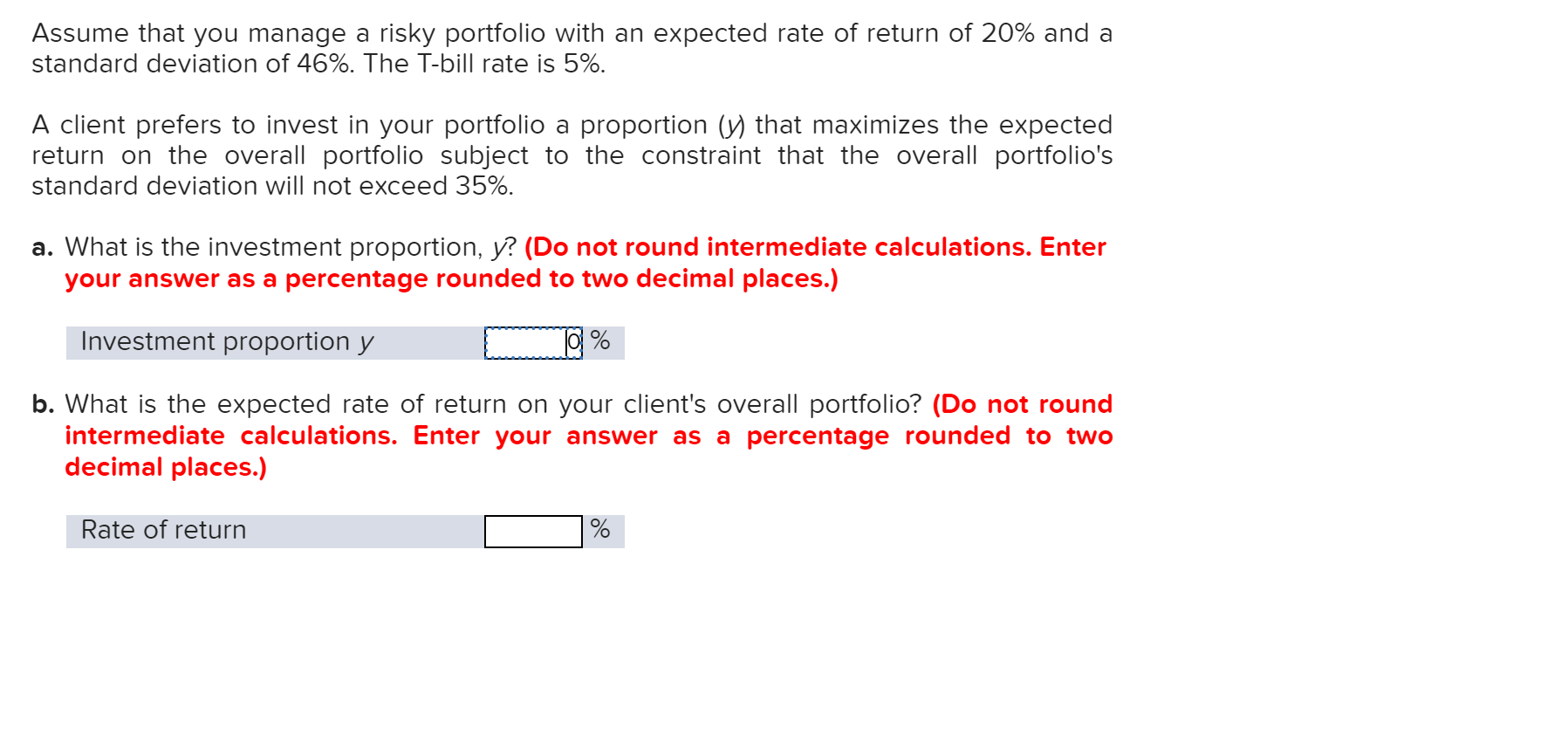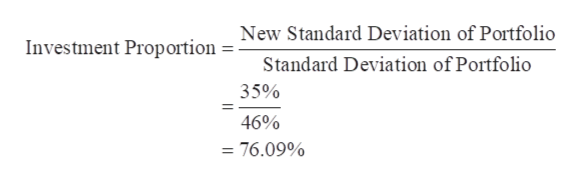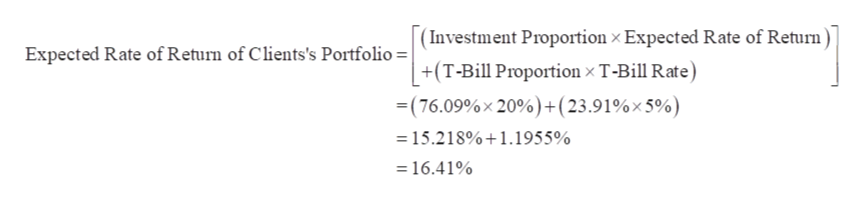# Assume that you manage a risky portfolio with an expected rate of return of 20% and astandard deviation of 46%. The T-bill rate is 5%.A client prefers to invest in your portfolio a proportion (y) that maximizes the expectedreturn on the overall portfolio subject to the constraint that the overall portfolio'sstandard deviation will not exceed 35%a. What is the investment proportion, y? (Do not round intermediate calculations. Enteryour answer as a percentage rounded to two decimal places.)Investment proportion yb. What is the expected raintermediate calculations. Enter your answer as a percentage rounded to twodecimal places.)of return on your client's overall portfolio? (Do not rounRate of return

Questionhelp_outlineImage TranscriptioncloseAssume that you manage a risky portfolio with an expected rate of return of 20% and a standard deviation of 46%. The T-bill rate is 5%. A client prefers to invest in your portfolio a proportion (y) that maximizes the expected return on the overall portfolio subject to the constraint that the overall portfolio's standard deviation will not exceed 35% a. What is the investment proportion, y? (Do not round intermediate calculations. Enter your answer as a percentage rounded to two decimal places.) Investment proportion y b. What is the expected ra intermediate calculations. Enter your answer as a percentage rounded to two decimal places.) of return on your client's overall portfolio? (Do not roun Rate of return fullscreen
check_circleExpert Solution
Step 1

a.

Given,

Standard Deviation of Portfolio = 46%

New Standard Deviation of Portfolio = 35%

Calculation of Investment Proportion y:help_outlineImage TranscriptioncloseNew Standard Deviation of Portfolio Investment Proportion Standard Deviation of Portfolio 35% 46% = 76.09% fullscreen
Step 2

b.

Investment Proportion = 76.09%

Expected Rate of Return = 20%

T-Bill Proportion = 23.91%

T-Bill Rate = 5%

Calculation of Expected Rate of Return of Client’s Portfolio:help_outlineImage Transcriptionclose(Investment Proportion x Expected Rate of Return) Expected Rate of Return of Clients's Portfolio = | +(T-Bill Proportion x T-Bill Rate) (76.09%x20%)+(23.91%x 5%) 15.218%+1.1955% =16.41% fullscreen
Step 3

Working Note:

Calculation of T-Bill Proport...

### Want to see the full answer?

See Solution

#### Want to see this answer and more?

Solutions are written by subject experts who are available 24/7. Questions are typically answered within 1 hour*

See Solution
*Response times may vary by subject and question
Tagged in